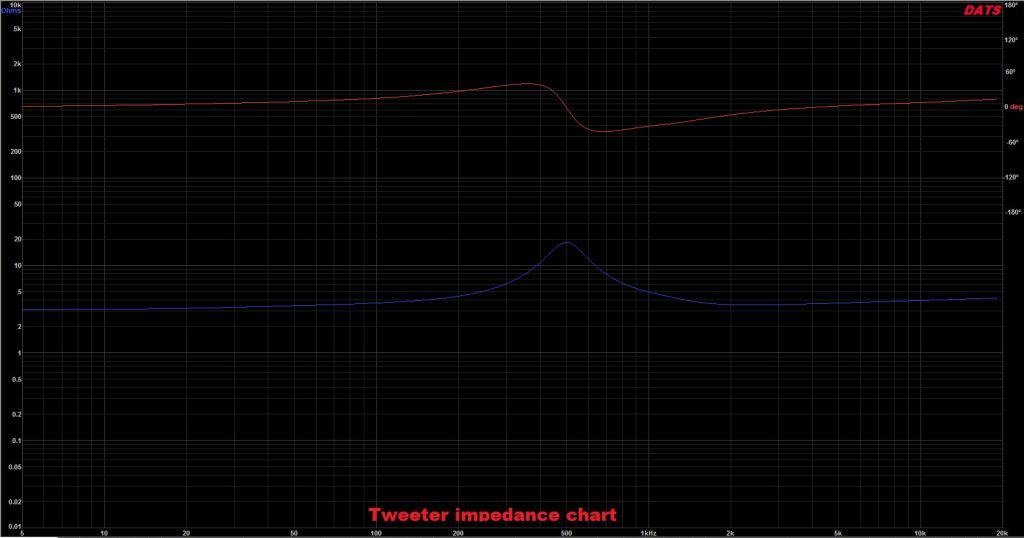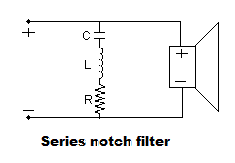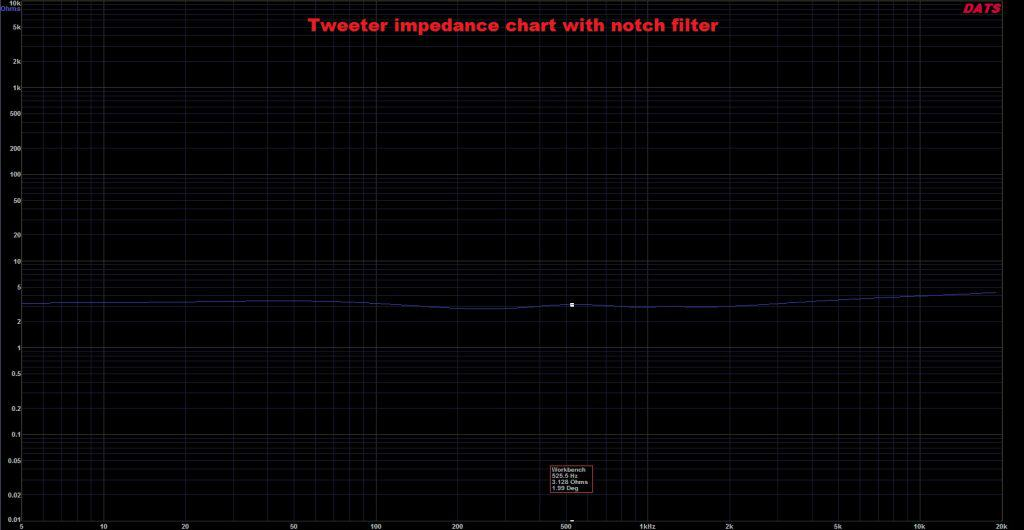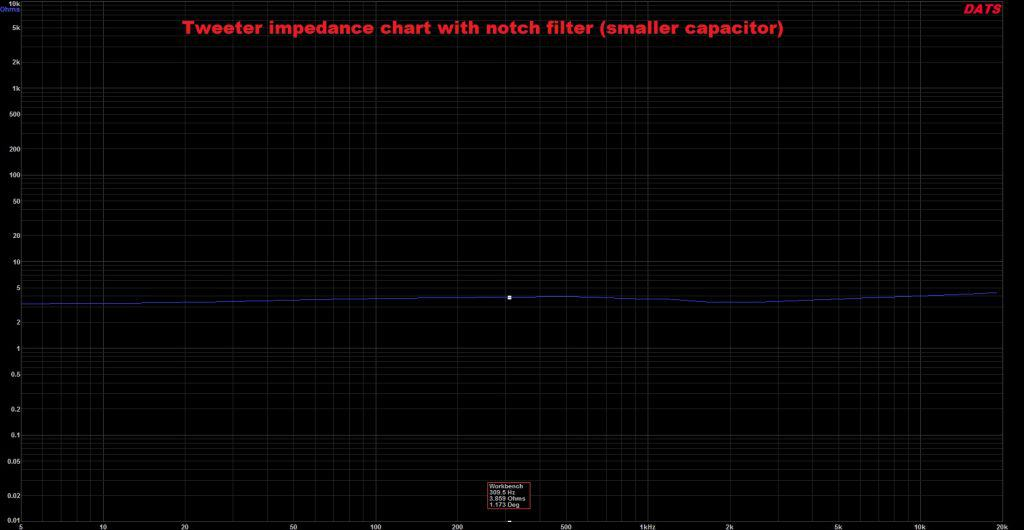## How to flatten impedance curve using a notch filter design?

A notch filter design is particularly useful when flattening the impedance of a tweeter. When it comes to speakers, there are two impedance rises : one is at resonance and the other is a slow rise due to voice coil inductance (as frequency increases). If we are talking about tweeters, the only thing that bothers us is the peak in impedance at resonance (because that is in the frequency band we want to filter). To reduce that impedance peak we have 2 options :

• A notch filter design.
• A shunt resistor.

Using a resistor in parallel with the tweeter will reduce the overall impedance by a small degree and the peak by a large degree. However, sometimes the peak is so large that the resistor is not enough, and a notch filter is in order. However, this may increase the cost of the crossover.

### Shunt resistor

Let’s quickly discuss the shunt resistor. This is the easy an cheap way of dealing with impedance spikes. Simply place a resistor in parallel with the tweeter and that’s it.

We are going to take a practical approach using a Vifa XT25TG30-04 tweeter. (Amazon affiliate paid link)We can see that the impedance peak at resonance is pretty high. Now we have to add a resistor in parallel with the tweeter to reduce the peak. We will start with a 10 ohm resistor and decrease its value till we get a satisfactory result.

As you can see, using a 1.5 Ohm resistor, has drastically reduced our peak impedance at resonance. However, it has also reduced our nominal impedance as well. Judging by the graph, the nominal impedance is now 1.1 Ohms. While the shunt resistor did the trick, the impedance is too low. A load of just 1 ohm will stress most amplifiers. This means we should try a notch filter design.

### Notch filter design

There are 2 types of notch filters : series and parallel. For our example we are discussing the series notch filter design. The notch filter contains the following components :

• a capacitor.
• an inductor.
• a resistor.

For this reason it is also called an LCR or RLC circuit.

To calculate the values of the components you will need the following parameters:

• Re – DC resistance.
• Qes – Electrical Q factor.
• Qms – Mechanical Q factor.
• fs – resonant frequency.

Then, you can calculate the values of the capacitor (C), inductor (L) and resistor (R) :

• C = 0.1592 / Re * Qes * fs
• L = 0.1592 * Qes * Re / fs
• R = Re + (Qes * Re / Qms)

### Practical example for our Vifa tweeter

Since I have not burned-in my tweeter, I will not measure the parameters. Instead, I will use those provided by the manufacturer :

• fs = 436 Hz
• Qes = 0.54
• Qms = 2.5
• Re = 3 Ohms

### How to design loudspeakers - video courses

Now let’s use these numbers to calculate the values of the components of our notch filter design.

• C = 0.1592 / 3 * 0.54 * 436 =0.1592 / 706.32 = 0.000225 = 225 μF
• L = 0.1592 * 0.54 * 3 / 436 = 0.2579 / 436 = 0.000592 = 0.59 mH
• R = 3 + (0.54 * 3 / 2.5) = 3 + 0.648 = 3.65 Ohm

When we apply the notch filter to our tweeter the impedance is changed to this :As you can see, the impedance is nice in flat. At least to the point where the variation of impedance is very little. There is one problem with this notch filter. The 225 μF capacitor. It is hugely expensive and it even exceeds the price of the tweeter .In conclusion, we are going to try to fit a smaller value capacitor and alter the coil and resistor to get a similar result. After some fiddling, these numbers came up :

• Capacitor : 100 μF
• Inductor : 1 mH
• Resistor : 4.6 OhmsTo be honest, the impedance curve is even flatter now and the capacitor is lower in value. The inductor is larger but, the prices of capacitors are much more significant.

The inductor has a resistance as well, so make sure you subtract from the value of the resistor the resistance of the inductor. In my case the inductor had a resistance of 0.7 Ohms. In conclusion, I used a 3.9 Ohm resistor (not a 4.6 Ohm).

### Conclusion

Flattening the impedance curve of a particular speaker is always a good starting point, when creating a high quality crossover. When it comes to tweeters, it’s always best to try a shunt resistor first. This will bring the cost of the crossover significantly lower. However, if all else fails, a notch filter will do the trick for any unfriendly tweeter.

#### References

1. Loudspeaker Design Cookbook 7th Edition by Vance Dickason (Audio Amateur Pubns, 2005). (Amazon affiliate paid link)